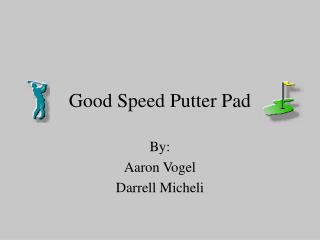DownloadDownload PresentationDownload Presentation- - - - - - - - - - - - - - - - - - - - - - - - - - - E N D - - - - - - - - - - - - - - - - - - - - - - - - - - -
##### Presentation Transcript

1. Good Speed Putter Pad By: Aaron Vogel Darrell Micheli

2. Overview of Project • Motivation for the Good Speed Putter Pad • Basic schematic of design • How did we break up the work?

3. Hardware Components • 3 Manual Switches • AML11 Series Lighted Solid-State Pushbutton Display Switch • 4 Seven Segment Displays • LSD3211-11 • 4 BCD Display Setting chips • CD4055BE • 4 sensors • HOA2498 series consisting of infrared emitting diode and a photodarlington

4. Sensors • Schematics • Response Time vs. Distance to Reflective Surface • Generated Waveform • Spacing • Integral to calculation of initial putter velocity

5. Derivation of Physics Equations • Refer to equations handout • Where may error be introduced? x = [ (2*vpi2) / µg*(1 + 2(mb/mp) + (mb2/mp2)) ]

6. Error Analysis • Sources of error • Calculation of coefficient of friction • Implemented calibration device • Calculation of initial putter velocity • Response time of sensors • Elapsed Time Error noted in demos • How was error analysis performed? • Explain excel spreadsheets

7. Error Analysis of Response Time

8. Error Analysis of Coefficient of Friction

9. Error Analysis of Response Time and Coefficient of Friction

10. Microcontroller Code START INITIALIZATION INTERRUPT? NO YES ISR

11. ISR FIRST OCCURANCE NO MATH1 YES STORE TIME INTO FIRST

12. MATH1 FIND TIME DIFFERENCE DIVIDE DIFF. BY 10 MULTIPLY BY 2 BLOCK CODE

13. BLOCK CODE FIND BLOCK DIFFERENCE BUTTONS STORE ELAPSED TIME MULTIPLY BY 10 DIVIDE BY A BLOCK AVERAGE ADD BLOCK STANDARD

14. BUTTONS BUTTON PUSHED NEXT BUTTON OR ERROR NO YES LOAD BUTTON MU MATH2

15. MATH2 LOAD ELAPSED TIME DECIMAL FT DIVIDE MU BY ELAPSED STORE VALUE INTO DECIMAL STORE VALUE INTO WHOLE DIVIDE REMAINDER BY ELAPSED

16. DECIMAL FT LOAD DECIMAL VALUE FIND DECIMAL BCD VALUE STORE BCD DECIMAL FEET

17. FEET LOAD WHOLE VALUE FIND BCD VALUE STORE BCD VALUE FINAL

18. FINAL ADD BCD FEET TO BCD DECIMAL OUTPUT ISR

19. Future Improvements • Trajectory sensors • Incline parameters • Adjustable coefficients of friction • Magnetic sensors • Graphic output display with appropriate scale

20. Questions???